# Get Homework Help With Chegg Study | Chegg.com PHY002-2019spr Maoging HW09_Magnetism Problem 26.34 1 of 10> Constants I Periodic Table PartA Determine the magnitudes and directions of the currents...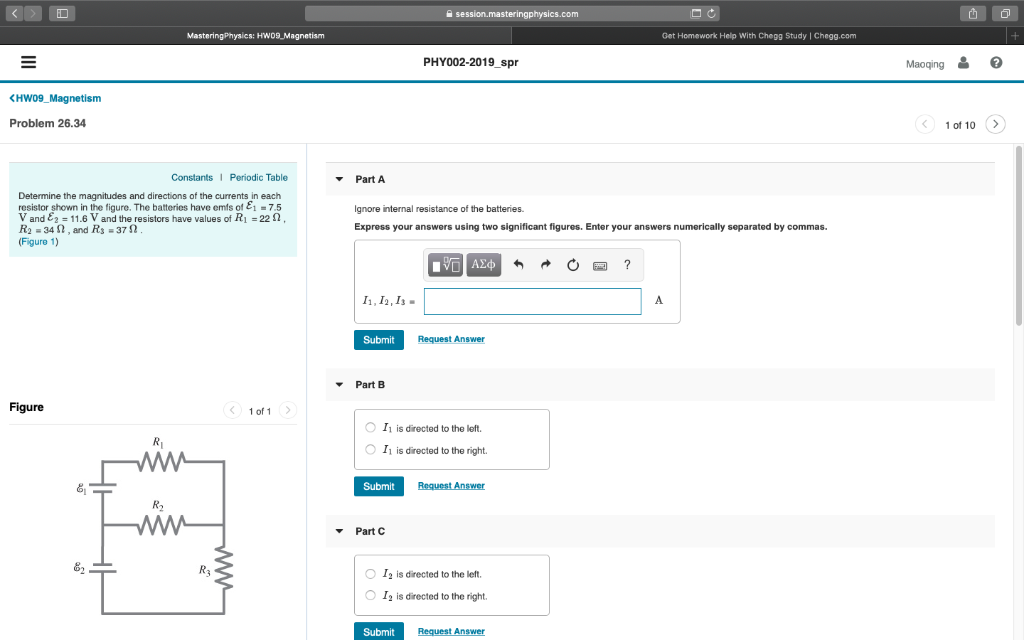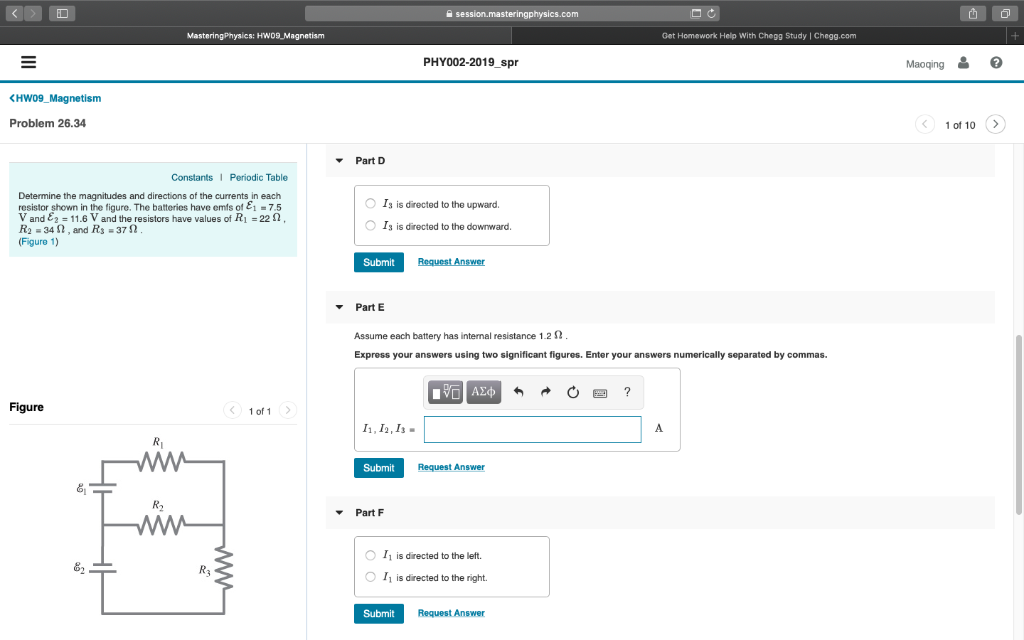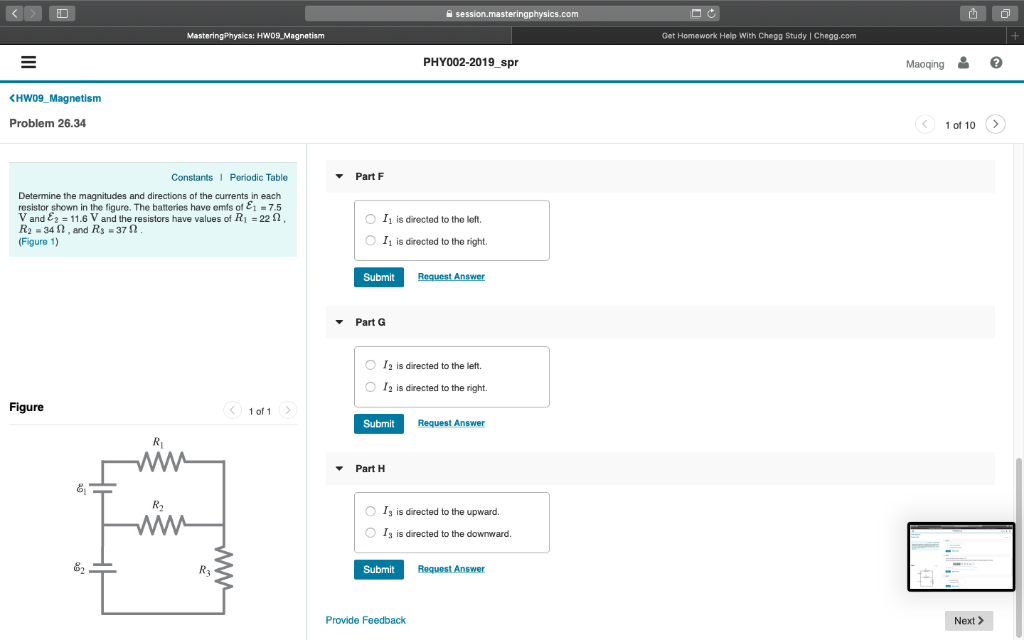Get Homework Help With Chegg Study | Chegg.com PHY002-2019spr Maoging HW09_Magnetism Problem 26.34 1 of 10> Constants I Periodic Table PartA Determine the magnitudes and directions of the currents in each resistor shown in the figure. The batteries have emfs of E1-7.5 V and E2 11.6 V and the resistors have values of R1 22 Ω R2 -34 2, and Rs-372 Figure 1) Ignore internal resistance of the batteries. Express your answers using two significant figures. Enter your answers numerically separated by commas. I1, I, Is Submit Request Answer Part B Figure 1 of 1 O I is directed to the left. O I is directed to the right Submit 81 R2 Part C O I2 is directed to the left I2 is directed to the right. O Submit
Get Homework Help With Chegg Study | Chegg.com PHY002-2019spr Maoqing HW09_Magnetism Problem 26.34 1 of 10> Part D Constants I Periodic Table Determine the magnitudes and directions of the currents in each resistor shown in the figure. The batteries have emfs of E1-7.5 V and E2 11.6 V and the resistors have values of R1 22 Ω R2=34 Ω , and R3=37 Ω Figure 1) O Is is directed to the upward. Is is directed to the downward O Submit Part E Assume each battery has internal resistance 1.2 Ω Express your answers using two significant figures. Enter your answers numerically separated by commas. Figure 1 of 1 I1, I, Is Submit Request Answer 81 R2 Part F O I1 is directed to the left. 11 is directed to the right. O Request Answer Submit
Get Homework Help With Chegg Study | Chegg.com PHY002-2019spr Maoqing Part F Constants I Periodic Table Determine the magnitudes and directions of the currents in each resistor shown in the figure. The batteries have emfs of E1-7.5 V and E2 11.6 V and the resistors have values of R1 22 Ω R2 -34 2, and Rs-372 Figure 1) O I1 is directed to the left. O I1 is directed to the right Submit Part G O I2 is directed to the left. O I2 is directed to the right. Figure 1 of 1 Submit Request Answer Part H 81 R2 O Is is directed to the upward. Is is directed to the downward. O Request Answer Submit Provide Feedback Next >

The current in circuit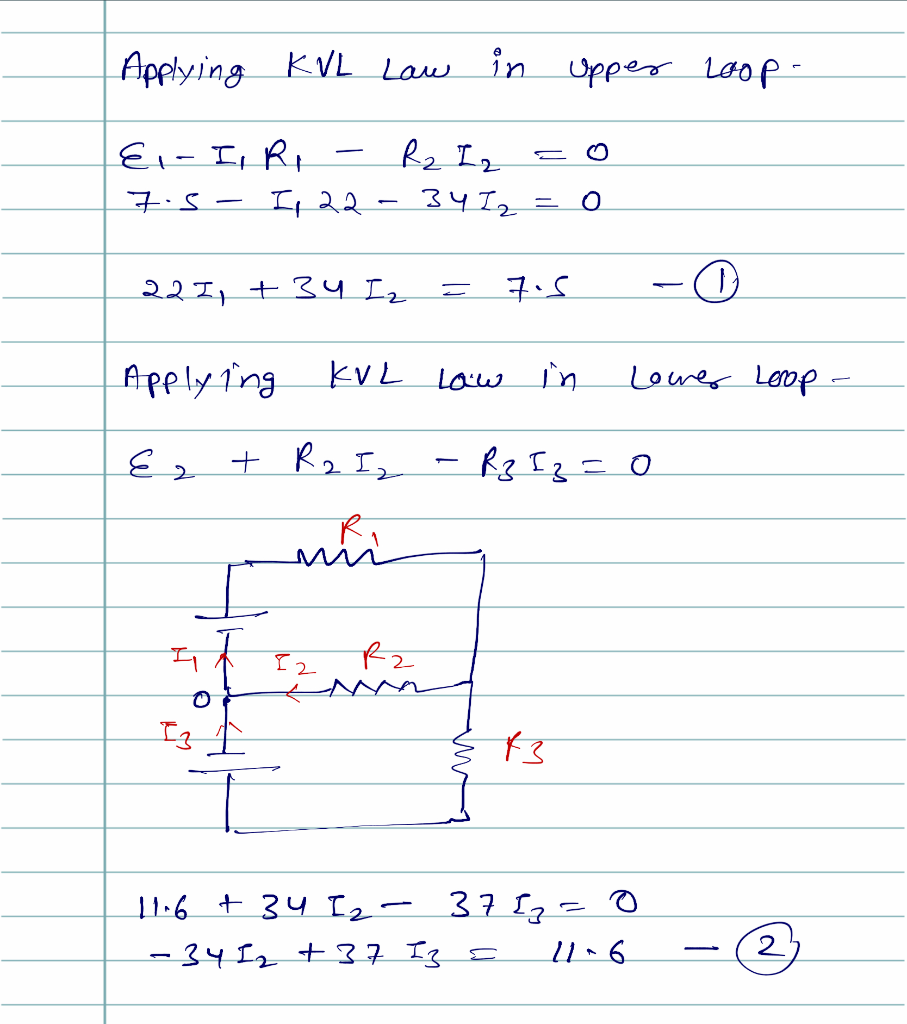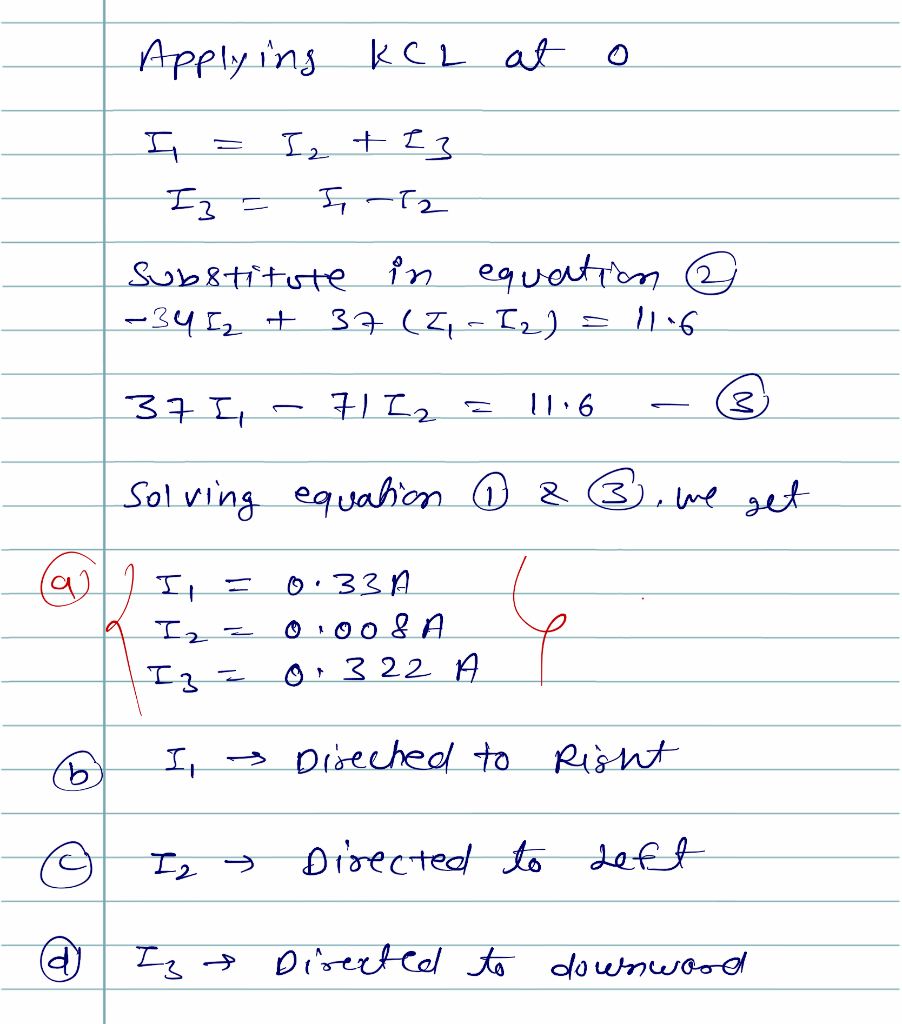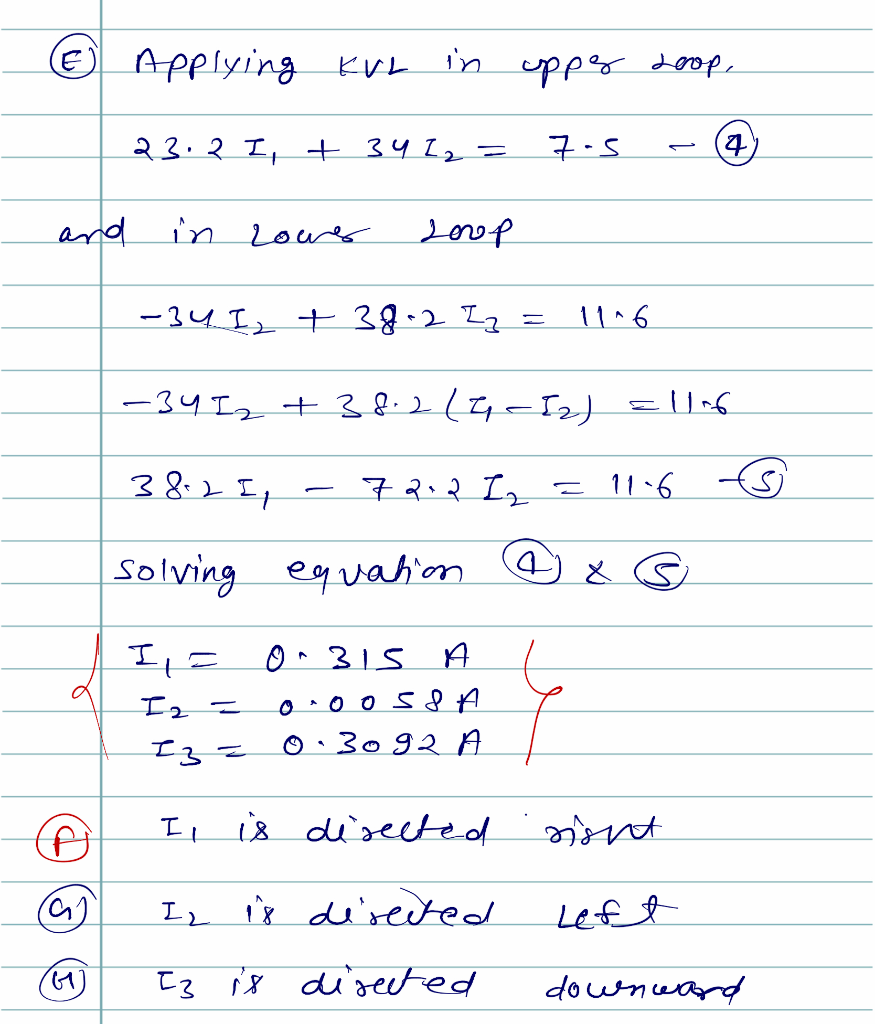##### Add Answer of: Get Homework Help With Chegg Study | Chegg.com PHY002-2019spr Maoging HW09_Magnetism Problem 26.34 1 of 10> Constants I Periodic Table PartA Determine the magnitudes and directions of the currents...
Similar Homework Help Questions
• ### Magnitude of Currents in ResistorsPart A:Determine the magnitudes of the currents in each resistor shown in the figure . The batteries have emfs of E1= 9.0 V and E2 = 12.0 V and the resistors havevalues of R1=22 ohm, R2=14 ohm, and R3-40 ohm. Enter your answers separated by commas. Express your answer using two significant figures.http://img543.imageshack.us/i/giancolich19p29.jpg/Part B: Determine the directions of the currents in each resistor.(a) right, left, up(b) left, right, down(c) left, right, up(d) right, left, down

• ### Physics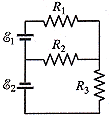Determine the magnitudes and directions of the currents in each resistor shown in Fig. 19-48. The batteries have emfs of script e1 = 9.0 V and script e2 = 13.0 V andthe resistors have values of R1 = 15 ohms, R2 = 20 ohms, and R3 = 28 ohms. Assume each battery has an internal resistance of 1.0 ohms.IR1?Ato the rightIR2?Ato the leftIR3?Atoward the top of the pageFigure 19-48

• ### I need help please, I calculated IR2 and the directions but the other ones I can't get them.

Determine the magnitudes and directions of the currents in each resistor shown in Fig. 19-48. The batteries have emfs of 1 = 9.0 V and 2 = 14.0 V and the resistorshave values of R1 = 15 , R2 = 20 , and R3 = 29 .IR1= _______ To the rightIR2= .3582 A To the leftIR3= _______ To the top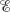Okay so here is the problem:Determine the magnitudes and directions of the currents ineach resistor shown in Fig. 19-48. The batteries have emfs of1 = 9.0 V and 2 =13.0V and the resistors have valuesof R1 = 15 ,R2 = 20, andR3 = 28 .IR1 A---Select---to the rightto the leftIR2 A---Select---to the rightto the leftIR3 A---Select---toward the top of the pagetoward the bottom of the pageFigure 19-48To get to the .286 I got the following formula first:-I1+ 3I2=9/15 (or .6)For...

• ### Help with parallel problemDetermine the magnitudes and directions of the currents in eachresistor shown in Fig. 19-48. The batteries have emfs of 1 =9.0 V and 2 = 17.0 Vand theresistors have values of R1 = 15 ,R2 = 20 , andR3 = 33 .IR10.088 A---Select---to the rightto the leftIR2 A---Select---to the rightto the leftIR30.292 A---Select---toward the bottom of the pagetoward the top of thepageFigure 19-48

• ### Part A Determine the magnitudes of the currents through R, and Rin (Figure 1), assuming that...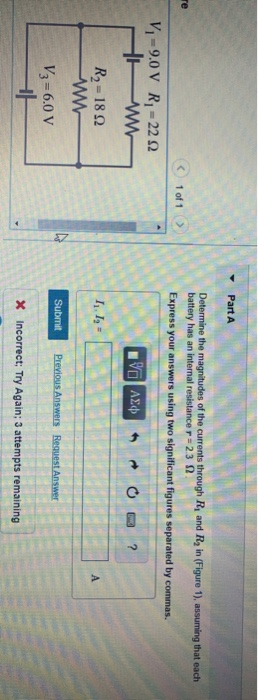Part A Determine the magnitudes of the currents through R, and Rin (Figure 1), assuming that each battery has an internal resistance r = 23 2 Express your answers using two significant figures separated by commas. 1 of 1 Vi=9.0 v R; = 2222 HAW R2 = 182 0 AED Submit Previous Answers Request Answer V3=6.0 V X Incorrect; Try Again; 3 attempts remaining

• ### Problem 21.61 Review PartA Consider the circuit shown in the figure(Figure 1). Suppose the four resistors...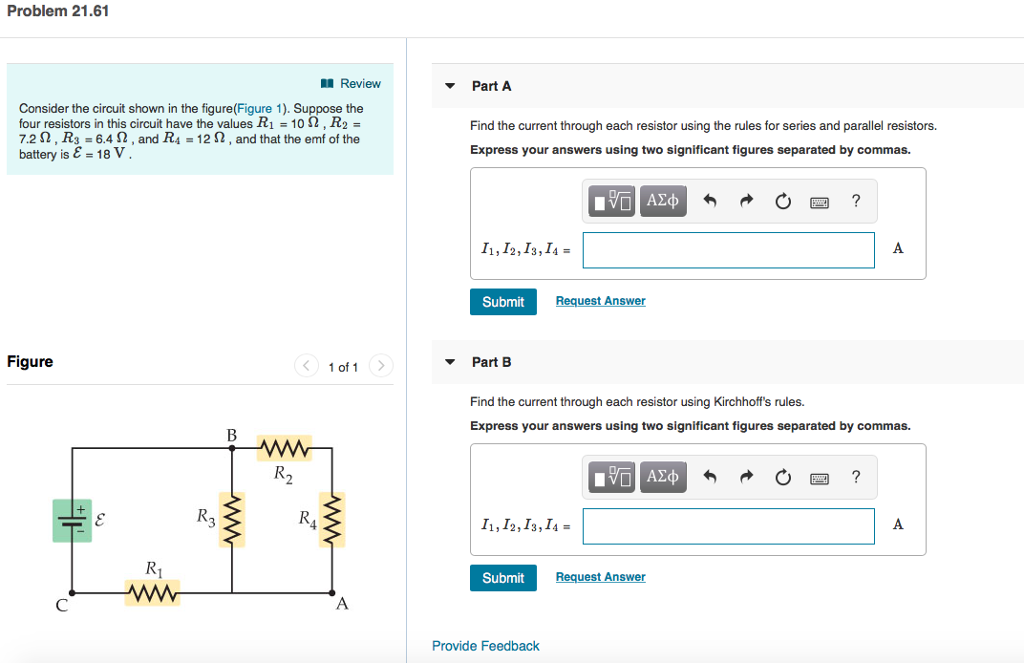Problem 21.61 Review PartA Consider the circuit shown in the figure(Figure 1). Suppose the four resistors in this circuit have the values R-10 Ω , R 7.2 S2, R3 6.42, and R4 12 2, and that the emf of the battery is E = 18 V . Find the current through each resistor using the rules for series and parallel resistors. Express your answers using two significant figures separated by commas. I1, I2,I3, I4- Submit Request Answer Figure 1 of...

• ### Problem 30.50 Part G Constants An inductor with inductance L 0.400 H and negligible resistance is connected to a batter...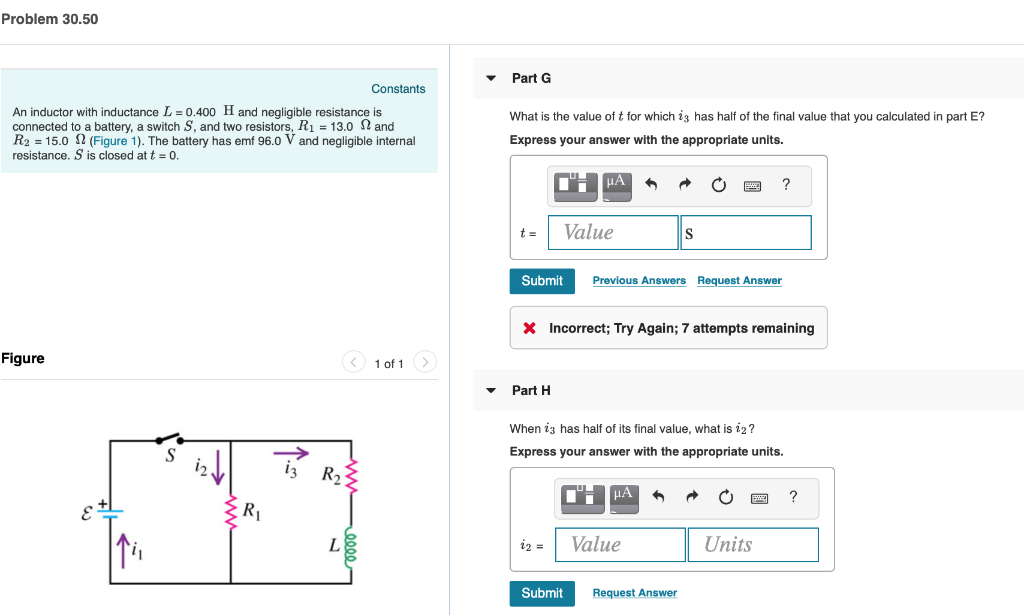Problem 30.50 Part G Constants An inductor with inductance L 0.400 H and negligible resistance is connected to a battery, a switch S, and two resistors, R1 13.0 2 and R2 15.0 2 (Figure 1). The battery has emf 96.0 V and negligible internal resistance. S is closed at t 0 What is the value of t for which is has half of the final value that you calculated in part E? Express your answer with the appropriate units. HA...

• ### Consider the circuit in the figure, with the current directions defined as shown Class Management Help...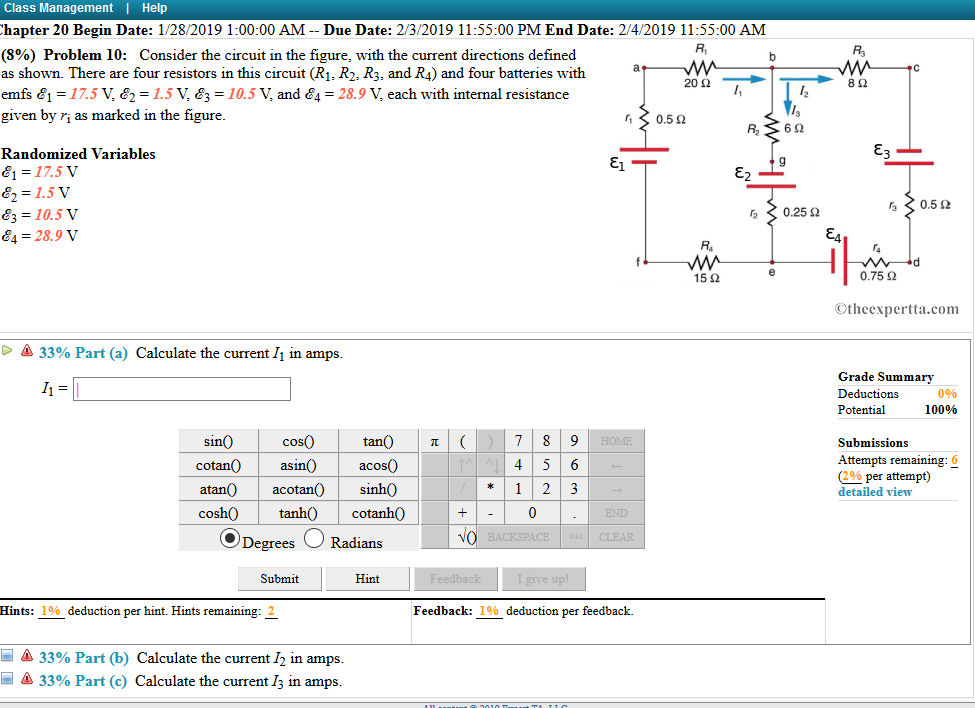Consider the circuit in the figure, with the current directions defined as shown Class Management Help hapter 20 Begin Date: 1/28/2019 1:00:00 AM - -Due Date: 2/3/2019 11:55:00 PM End Date: 2/4/2019 11:55:00 AM (896) Problem 10: Consider the circuit in the figure, with the current directions defined as shown. There are four resistors in this circuit (R1, R2, R3, and R4) and four batteries with emfs 1- 17.5 V, &1.5 V, 83-10.5 V, and &428.9 V, each with internal...

• ### Review I Constants 1 Periodic Table Consider the following curve (Figure 1) for the titration of...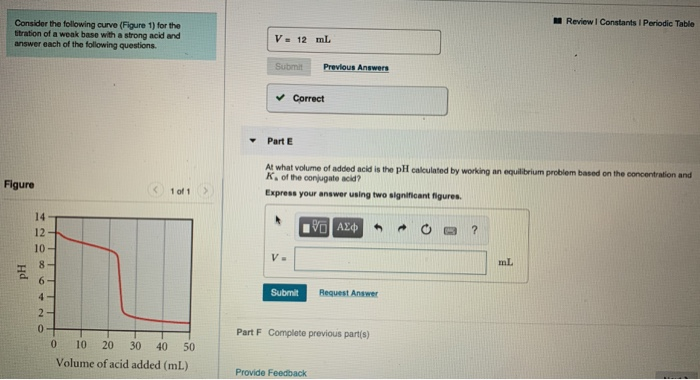Review I Constants 1 Periodic Table Consider the following curve (Figure 1) for the titration of a weak base with a strong acid and answer each of the following questions. V = 12 mL Submit Previous Answers Correct Part E Figure At what volume of added acid is the pH calculated by working an equilibrium problem based on the concentration and k of the conjugate acid? Express your answer using two significant figures 1 of 1 > 10 AED -...

Need Online Homework Help?